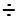Fractions: Subtracting with Unlike Denominators

 Remember! When the DENOMINATORS (number at bottom) are different, you must use multiplication to change them into the same denominators by finding common multiples. Then multiply the NUMERATOR (number at top) by the same number. Then just subtract the numerators. Simplify with division if necessary. Remember! Whatever you do to the top, do to the bottom. Name ________________________________ Date ________________________________ Time: ________________________________
Example 1
Example 2

 1. 3 x2 = 6 ___ ____ 5 x2 = 10 2 = 2 - _____ ____ 10 = 10
 42 = 2 ____ ____ 102 = 5
 2. 3 x3 = 9 ___ ____ 4 x3 = 12 2 x4 = 8 - ___ ____ 3 x4 = 12
 1 ____ 12
 3. 5 _____ 6 2 - _____ 3

 4. 2 _____ 6 1 - _____ 3

 5. 3 _____ 10 1 - _____ 5

 6. 3 _____ 5 1 - _____ 3

 7. 5 _____ 7 1 - _____ 3

 8. 7 _____ 8 3 - _____ 4

 9. 4 _____ 5 2 - _____ 4

 10. 3 _____ 6 1 - _____ 4

 11. 4 _____ 6 1 - _____ 3

 12. 1 _____ 2 2 - _____ 6

 13. 2 _____ 3 2 - _____ 12

 14. 7 _____ 9 1 - _____ 3

 15. 5 _____ 6 2 - _____ 5

 16. 5 _____ 8 2 - _____ 4

 17. 2 _____ 3 1 - _____ 9

 18. 5 _____ 7 2 - _____ 14